# The Gamma Of Levered ETFs

Levered ETFs use derivatives to amplify the return of an underlying index. Here’s a list of 2x levered ETFs. For example, QLD gives you 2x the return of QQQ (Nasdaq 100). Levered ETFs use derivatives to get the levered exposure. In this post, we will compute the delta and gamma of levered ETFs and what that means for investors and traders.

## Levered ETF Delta

In options, delta is the sensitivity of the option premium to a change in the underlying stock. If you own a 50% delta call and the stock price goes up by \$1, you make \$.50. If the stock went down \$1, you lost \$.50. Delta, generally speaking, is a rate of change of p/l with respect to how some asset moves. I like to say it’s the slope of your p/l based on how the reference asset changes.

For levered ETFs, the delta is simply the leverage factor. If you buy QLD, the 2x version of QQQ, you get 2x the return of QQQ. So if QQQ is up 1%, you earn 2%. If QQQ is down 1%, you lose 2%. If you invest \$1,000 in QLD your p/l acts as if you had invested \$2,000.

\$100 worth of QLD is the equivalent exposure of \$200 of QQQ.

Your dollar delta is \$200 with respect to QQQ. If QQQ goes up 1%, you make 1% * \$200 QQQ deltas = \$2

The extra exposure cuts both ways. On down days, you will lose 2x what the underlying QQQ index returns.

The takeaway is that your position or delta is 2x the underlying exposure.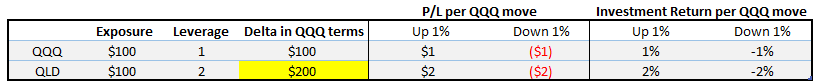Dollar delta of levered ETF = Exposure x Leverage Factor

In this case, QLD dollar delta is \$200 (\$100 x 2).

Note that QLD is a derivative with a QQQ underlyer.

## Levered ETF Gamma

QLD is a derivative because it “derives” its value from QQQ. \$100 exposure to QLD represents a \$200 exposure to QQQ. In practice, the ETF’s manager offers this levered exposure by engaging in a swap with a bank that guarantees the ETF’s assets will return the underlying index times the leverage factor. For the bank to offer such a swap, it must be able to manufacture that return in its own portfolio. So in the case of QLD, the bank simply buys 2x notional the NAV of QLD so that its delta or slope of p/l matches the ETFs promise.

So if the ETF has a NAV of \$1B, the bank must maintain exposure of \$2B QQQ deltas. That way, if QQQ goes up 10%, the bank makes \$200mm which it contributes to the ETF’s assets so the new NAV would be \$1.2B.

Notice what happened:

• QQQ rallied 10% (the reference index)
• QLD rallies 20% (the levered ETF’s NAV goes from\$1B –> \$1.2B)
• The bank’s initial QQQ delta of \$2B has increased to \$2.2B.

Uh oh.

To continue delivering 2x returns, the bank’s delta needs to be 2x the ETF’s assets or \$2.4B, but it’s only \$2.2B! The bank must buy \$200M worth of QQQ deltas (either via QQQs, Nasdaq futures, or the basket of stocks).

If we recall from options, gamma is the change in delta due to a change in stock price. The bank’s delta went from 2 (ie \$2B/\$1B) to 1.833 (\$2.2B/\$1.2B). So it got shorter deltas, in a rising market –> negative gamma!

The bank must dynamically rebalance its delta each day to maintain a delta of 2x the ETF’s assets. And the adjustment means it must buy deltas at the close of an up day in the market or sell deltas at the close of a down day. Levered ETFs, therefore, amplify price moves. The larger the daily move, the larger the rebalancing trades need to be!

I’ve covered this before in Levered ETF/ETN tool, where I give you this spreadsheet to compute the rebalancing trades: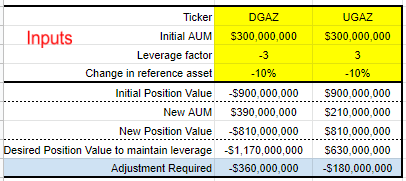## From Brute Force To Symbols

There was confusion on Twitter about how levered ETFs worked recently and professor @quantian stepped up:

Junior PM interview question: An X-times leveraged fund tracks an underlying asset S. After time T, S have moved to ST = (1+dS)S0. The initial delta is of course X. What is the portfolio gamma, defined as (dDelta)/(dS), as a function of X?

Despite correctly understanding how levered and inverse ETFs work I struggled to answer this question with a general solution (ie convert the computations we brute-forced above into math symbols). It turns out the solution is a short expression and worth deriving to find an elegant insight.

@quantian responded to my difficulty with the derivation.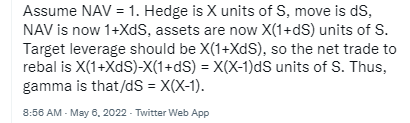I’ll walk you through that slowly.
Mapping variables to @quantian’s question:
• NAV =1

You are investing in a levered ETF that starts with a NAV of 1

• X = The leverage factor

The bank needs to have a delta of X to deliver the levered exposure. For a 2x ETF, the bank’s initial delta will be 2 * NAV = 2

• S = the underlying reference index

The dynamic:

• When S moves, the bank’s delta will no longer be exactly X times the NAV. Its delta changed as S changed. That’s the definition of gamma.
• When S moves, the bank needs to rebalance (buy or sell) units of S to maintain the desired delta of X. The rebalancing amount is therefore the change in delta or gamma.

Let’s find the general formula for the gamma (ie change in delta) in terms of X. Remember X is the leverage factor and therefore the bank’s desired delta.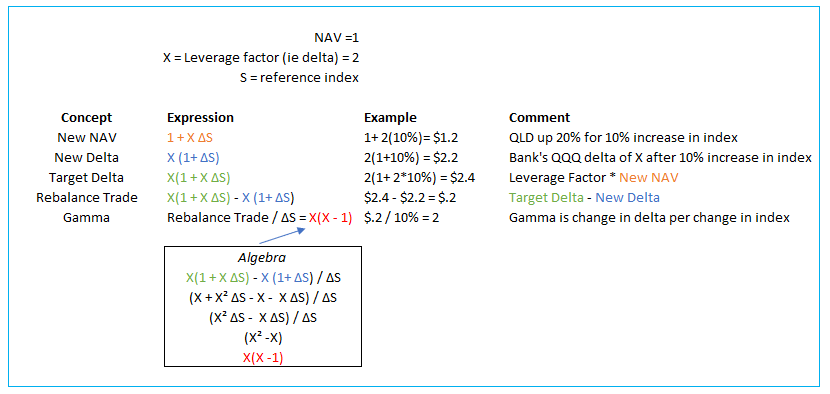The general formula for the gamma as a function of the change in the underlying index is, therefore:
X (X – 1)
where X = leverage factor
Intuition

There are 2 key insights when we look at this elegant expression:

1. The gamma, or imbalance in delta due to the move, is proportional to the square of the leverage factor. The more levered the ETF, the larger the delta adjustment required. If there was no leverage (like SPY to the SPX index), the gamma is 0 because 0 (0-1) = 0

2. The asymmetry of inverse ETFs — they require larger rebalances for the same size move! Imagine a simple inverse ETF with no leverage.

-1 (-1 – 1) = 2

A simple inverse ETF, has the same gamma as a double long ETF.

Consider how a double short ETF has a gamma of 6!:

-2 (-2 -1) = 6

When I admit that I had only figured out the rebalancing quantities by working out the mechanics by brute force in Excel, @quantian had a neat observation:

I originally found this by doing the brute force Excel approach! Then I plotted it and was like “hm, that’s just a parabola, I bet I could simplify this”

X2– X shows us that the gamma of an inverse ETF is equivalent to the gamma of its counterpart long of one degree higher. For example, a triple-short ETF has the same gamma as a 4x long. Or a simple inverse ETF has the gamma of a double long. The fact that a 1x inverse ETF has gamma at all is a clue to the difficulty of running a short book…when you win, your position size shrinks and the effect is compounded by the fact that your position is shrinking even faster relative to your growing AUM as your shorts profit!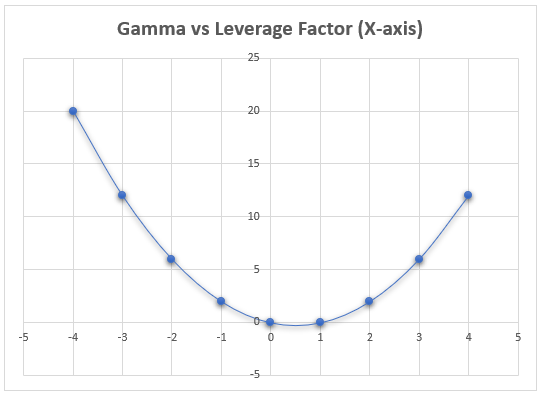I’ve explained this asymmetry before in The difficulty with shorting and inverse positions as well as the asymmetry of redemptions:

• As the reference asset rallies, position size gets bigger and AUM drops due to losses. As reference asset falls, position size shrinks while AUM increase due to profits.
• Redemptions can stabilize rebalance requirements in declines and exacerbate rebalance quantities in rallies as redemptions reduce shares outstanding and in turn AUM while in both cases triggering the fund’s need to buy the reference asset which again is stabilizing after declines but not after rallies. In other words, profit-taking is stabilizing while puking is de-stabilizing.

Rebalancing In Real Life

The amount of the rebalance from our derivation is:

X(1 + X ΔS) – X (1+ ΔS)

where:

X = leverage factor

ΔS = percent change in underlying index

Another way to write that is:

X (X-1) (ΔS)

In our example, 2 * (2-1) * 10% = \$.2 or an imbalance of 20% of the original NAV!

In practice, the size of the rebalance trade is of practical use. If an index is up or down a lot as you approach the end of a trading day then you can expect flows that exacerbate the move as levered ETFs must buy on up days and sell on down days to rebalance. It doesn’t matter if the ETF is long or inverse, the imbalance is always destabilizing in that it trades in the same direction as the move. The size of flows depends on how much AUM levered ETFs are holding but they can possibly be mitigated by profit-taking redemptions.

During the GFC, levered financial ETFs had large rebalance trades amidst all the volatility in bank stocks. Estimating, frontrunning, and trading against the rebalance to close was a popular game for traders who understood this dynamic. Years later levered mining ETFs saw similar behavior as precious metals came in focus in the aftermath of GFC stimulus. Levered energy ETFs, both in oil and natural gas, have ebbed and flowed in popularity. When they are in vogue, you can try to estimate the closing buy/sell imbalances that accompany highly volatile days.

Warning Label

Levered ETFs are trading tools that are not suitable for investing. They do a good job of matching the levered return of an underlying index intraday. The sum of all the negative gamma trading is expensive as the mechanical re-balancing gets front-run and “arbed” by traders. This creates significant drag on the levered ETF’s assets. In fact, if the borrowing costs to short levered ETFs were not punitive, a popular strategy would be to short both the long and short versions of the same ETF, allowing the neutral arbitrageur to harvest both the expense ratios and negative gamma costs from tracking the index!

ETFs such as USO or VXX which hold futures are famous for bleeding over time. That blood comes from periods when the underlying futures term structure is in contango and the corresponding negative “roll” returns (Campbell has a timeless paper decomposing spot and roll returns titled Deconstructing Futures Returns: The Role of Roll Yield). This is a separate issue from the negative gamma effect of levered or inverse ETFs.

Some ETFs combine all the misery into one simple ticker. SCO is a 2x-levered, inverse ETF referencing oil futures. These do not belong in buy-and-hold portfolios. Meth heads only please.

[The amount of variance drag that comes from levered ETFs depends on the path which makes the options especially tricky. I don’t explain how to price options on levered ETFs but this post is a clue to complication — Path: How Compounding Alters Return Distributions]

### Key Takeaways

• Levered ETFs are derivatives. Their delta changes as the underlying index moves. This change in delta is the definition of gamma.

• Levered and inverse ETFs have “negative gamma” in that they must always rebalance in a destabilizing manner — in the direction of the underlying move.
• The required rebalance in terms of the fund’s NAV is:

X (X-1) (ΔS)

• The size of the rebalance is proportional to the square of the leverage factor. The higher the leverage factor the larger the rebalance. For a given leverage factor, inverse ETFs have larger gammas.

• The drag that comes from levered ETFs means they will fail to track the desired exposure on long horizons. They are better suited to trading or short-term risk management.

### Appendix: Using Delta To Summarize Exposures

We can see that delta is not limited to options, but is a useful way to denote exposures in derivatives generally. It allows you to sum deltas that reference the same underlying to compute a net exposure to that underlying.

Consider a portfolio:

• Short 2000 shares of QQQ
• Long 1000 shares of QLD
• Long 50 1 month 53% delta calls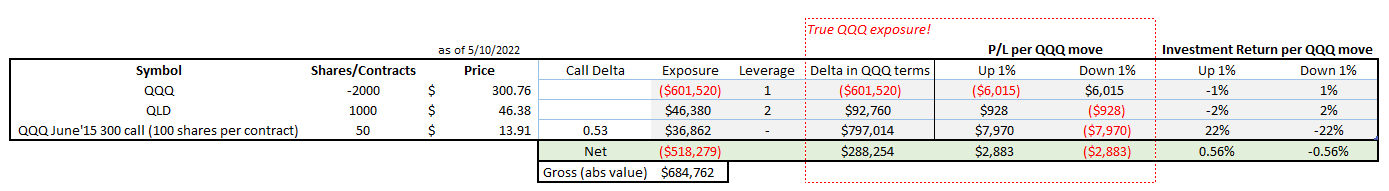By transforming exposures into deltas then collapsing them into a single number we can answer the question, “what’s my p/l if QQQ goes up 1%?”

We want to know the slope of our portfolio vis a vis QQQ.

A few observations:

• I computed net returns for the portfolio based on the gross (absolute value of exposures)
• The option exposure is just the premium, but what we really care about is the delta coming from the options. Even though the total premium is <\$37k, the largest delta is coming from the options position.